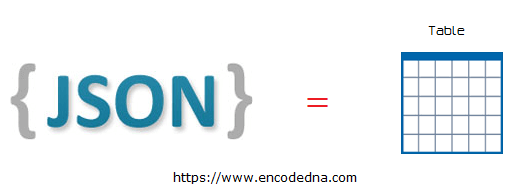﻿ Convert JSON Data Dynamically to HTML Table using JavaScript

# Convert JSON Data Dynamically to HTML Table using JavaScript

JSON or JavaScript Object Notation is a simple easy to understand data format. JSON is lightweight and language independent. Here, in this article I’ll show you how to convert JSON data to an HTML table using JavaScript. In addition, you will learn how you can create a table in JavaScript dynamically using createElement() Method.See this demo

Note: You can also use jQuery to convert data from a JSON file to an HTML table

## The JSON structure

The JSON for this example looks like this.

```[
{
"Book ID": "1",
"Book Name": "Computer Architecture",
"Category": "Computers",
"Price": "125.60"
},
{
"Book ID": "2",
"Book Name": "Asp.Net 4 Blue Book",
"Category": "Programming",
"Price": "56.00"
},
{
"Book ID": "3",
"Book Name": "Popular Science",
"Category": "Science",
"Price": "210.40"
}
]```

The above JSON has an array of three different Books with ID, Name, Category and Price. Just three records for the example. You can add more.

I want the program to read JSON data, get the columns (Book ID, Book Name etc.) for the <table> header, and the values for the respective headers.

The Markup and the Script

In the markup section, I have a button to call a JavaScript function, which will extract the JSON data from the array, create a <table> with header and rows dynamically and finally populate the data in it. I also have DIV element that will serve as a container for our table. After I populate the data, I’ll append the <table> to the <div>.

```<!DOCTYPE html>
<html>
<style>
table, th, td
{
border: solid 1px #ddd;
border-collapse: collapse;
text-align: center;
}
th {
font-weight:bold;
}
</style>
<body>
<input type='button' onclick='tableFromJson()' value='Create Table from JSON data' />
<p id="showData"></p>
</body>

<script>
let tableFromJson = () => {
// the json data.
const myBooks = [
{'Book ID': '1', 'Book Name': 'Challenging Times',
},
{'Book ID': '2', 'Book Name': 'Learn JavaScript',
'Category': 'Programming', 'Price': '56.00'
},
{'Book ID': '3', 'Book Name': 'Popular Science',
'Category': 'Science', 'Price': '210.40'
}
]

// Extract value from table header.
// ('Book ID', 'Book Name', 'Category' and 'Price')
let col = [];
for (let i = 0; i < myBooks.length; i++) {
for (let key in myBooks[i]) {
if (col.indexOf(key) === -1) {
col.push(key);
}
}
}

// Create table.
const table = document.createElement("table");

let tr = table.insertRow(-1);                   // table row.

for (let i = 0; i < col.length; i++) {
let th = document.createElement("th");      // table header.
th.innerHTML = col[i];
tr.appendChild(th);
}

// add json data to the table as rows.
for (let i = 0; i < myBooks.length; i++) {

tr = table.insertRow(-1);

for (let j = 0; j < col.length; j++) {
let tabCell = tr.insertCell(-1);
tabCell.innerHTML = myBooks[i][col[j]];
}
}

// Now, add the newly created table with json data, to a container.
const divShowData = document.getElementById('showData');
divShowData.innerHTML = "";
divShowData.appendChild(table);
}
</script>
</html>```

The JSON data that I have mentioned is stored in an array called myBooks. The structure is very simple and you can add more data to it.

First, it extracts values for the table's header. For that I have declared another array called var col = []. It will loop through each JSON and checks the first key index and store it in the array. See the output in your browsers console window.

```for (let i = 0; i < myBooks.length; i++) {
for (let key in myBooks[i]) {
col.push(key);
console.log (key);
}
}```

Next, it creates an <table> dynamically with a row for the header.

```const table = document.createElement("table");
let tr = table.insertRow(-1);       // Table row.```

In the first row of the table, I’ll create <th> (table header), assign values (from the array col []) for the header and append the <th> to the row.

```for (let i = 0; i < col.length; i++) {
let th = document.createElement("th");      // Table header.
th.innerHTML = col[i];
tr.appendChild(th);
}```

Finally, we will get the real values under each header, create cells for each table row and store the value in it.

`tabCell.innerHTML = myBooks[i][col[j]];    // Get book details for each header.`

It is like getting value from myBooks[i] of column col [j]. Remember array col [] has a list of unique headers.

See this demo

At the end, we are adding the newly created <table> to a container (a DIV element). That’s it. Hope you find this example useful. Thanks for reading.